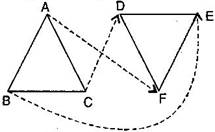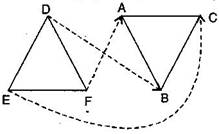# EXERCISE  7.1

QUESTION 1

Complete the following statements :

1. Two line segments are congruent if _______________.

2. Among two congruent angles, one has a measure of 70∘ , the measure of other angle is _______________.

3. When we write A = B, we actually mean _______________.

Sol :

(a) they have the same length

(b) 70∘

(c) m∠A = m∠B

QUESTION 2

Give any two real time examples for congruent shapes

Sol :

(i) Two footballs

(ii) Two teacher’s tables

QUESTION 3

If ΔABC≅ΔFED under the correspondence ABC FED , write all the corresponding congruent parts of the triangles.

Sol :

Given:  ΔABCΔFEDThe corresponding congruent parts of the triangles are:

(i) A F

(ii) E

(iii)  C D

(iv)(v)(vi)QUESTION 4

If ΔDEF≅ΔBCA, write the part(s) of ΔBCA that correspond to:

(i) E  (ii)(iii) F  (iv)Sol :

Given : ΔDEFΔBCA(i) C

(ii)(iii) A

(iv)Insert math as
$${}$$